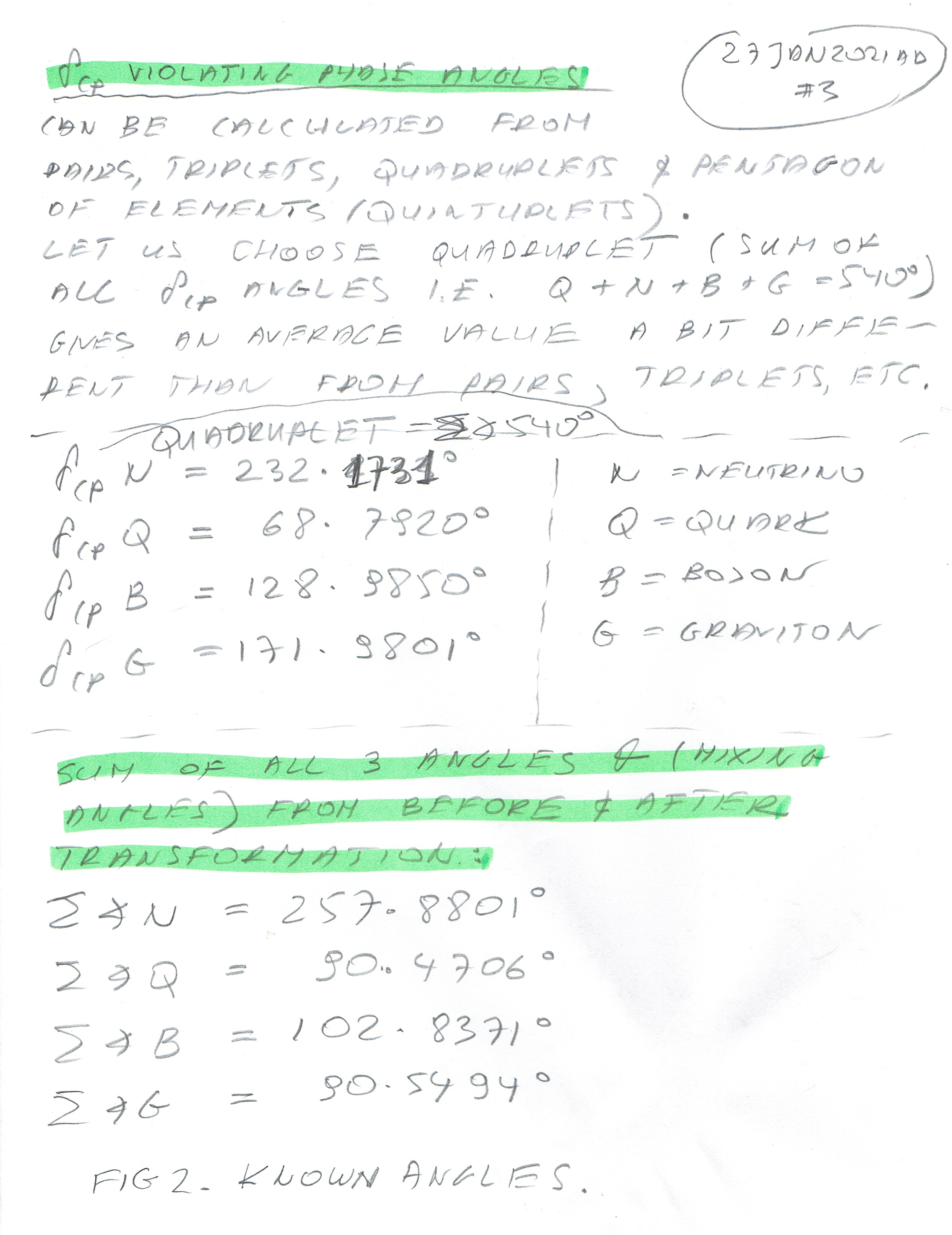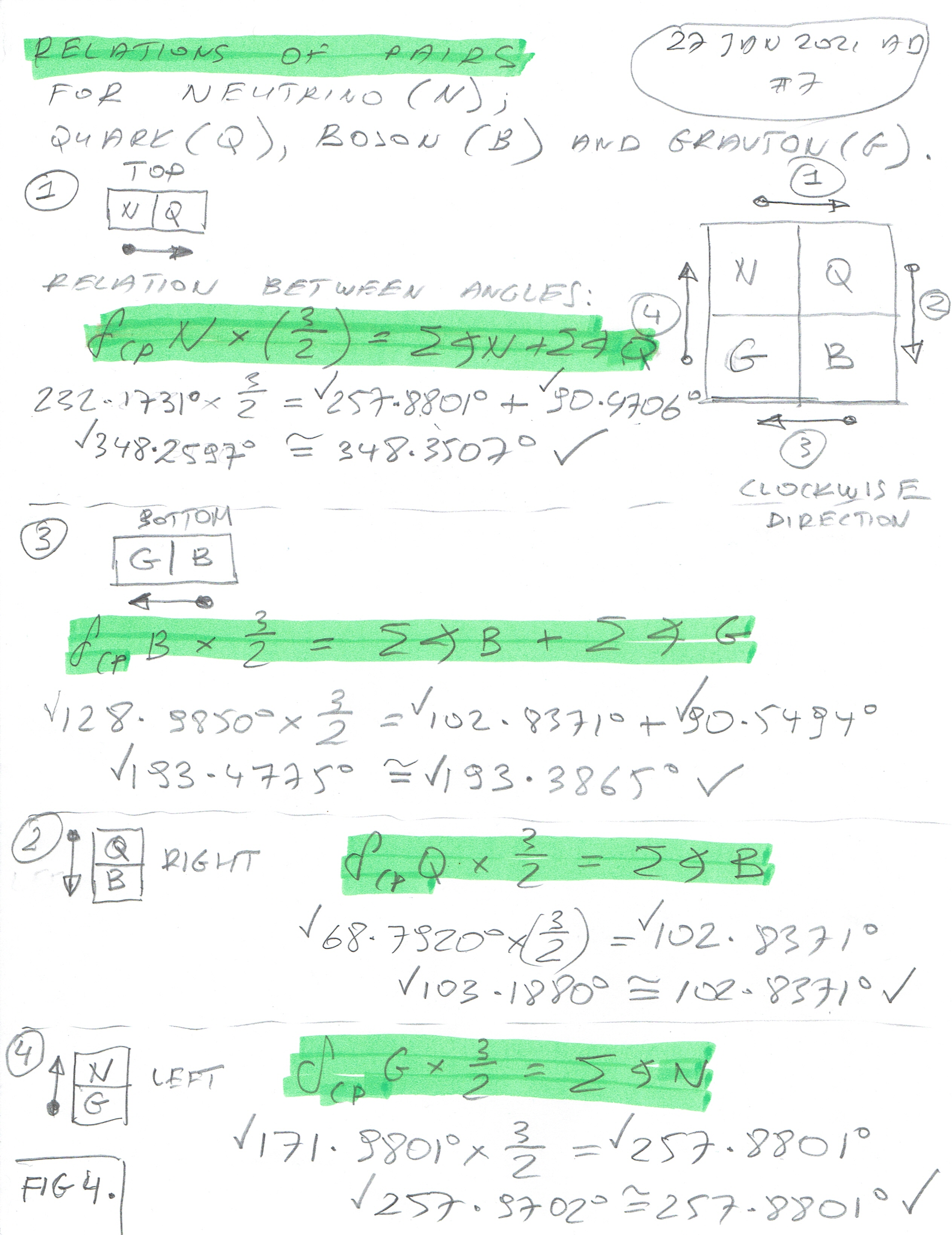There are simple formulas binding together Delta CP Violating Phase Angles and Sigma Mixing (Oscillation) Angles /sums from the three theta angles from before and after transform/. These formulas are derived first from the pairs of elements /Neutrino-Quark; Boson-Graviton; Quark-Boson; Graviton-Neutrino/.

In the following articles, I will extend the reasoning to triplets and quadruplets of elements (all relations between the angles).

There is no need for doubts, the four elements have the same rules as the following four elements i.e. Space, Time, Membrane Internal, and Membrane External.

There are two tests that can validate my claim (at the very end of the series).

All we need for now is the values of all four Delta CP Violating Phase Angles and all four Sigma Mixing (Oscillation) Angles. Those values were calculated before.

Here is a script with these results:

Figure 2. Known Angles:And, in the next script, there are those simple relations I was talking about.

1. Neutrino - Quark pair:

Delta CP Neutrino angle  X (3/2) = Sigma Neutrino angle + Sigma Quark angle

2. Boson - Graviton pair:

Delta CP Boson angle X (3/2) = Sigma Boson Angle + Sigma Graviton angle

3. Quark-Boson pair:

Delta CP Quark angle X (3/2) = Sigma Boson angle

4. Graviton - Neutrino pair:

Delta CP Graviton angle X (3/2) = Sigma Neutrino angle.

You can see it better in the following script:

Figure 4 - Relations between the angles of pairs of elements:These relations will let me arrive at the future articles at all eight Delta CP Violating Phase Angles and all eight Sigma Mixing (Oscillation) Angles (these angles are sums of all three Theta Mixing Angles from before transform and after the transform - which simply means the angles which were transformed into the positive real part of the plane).